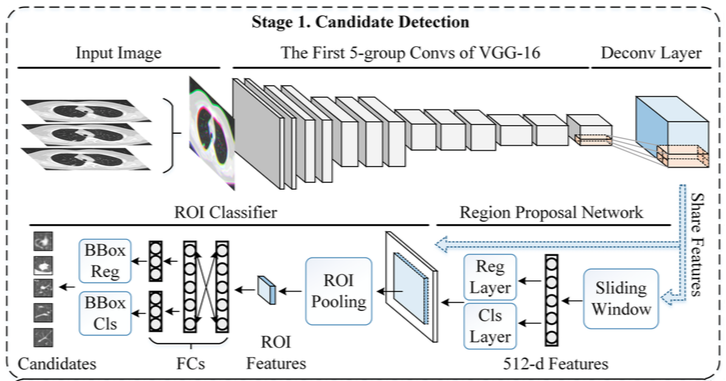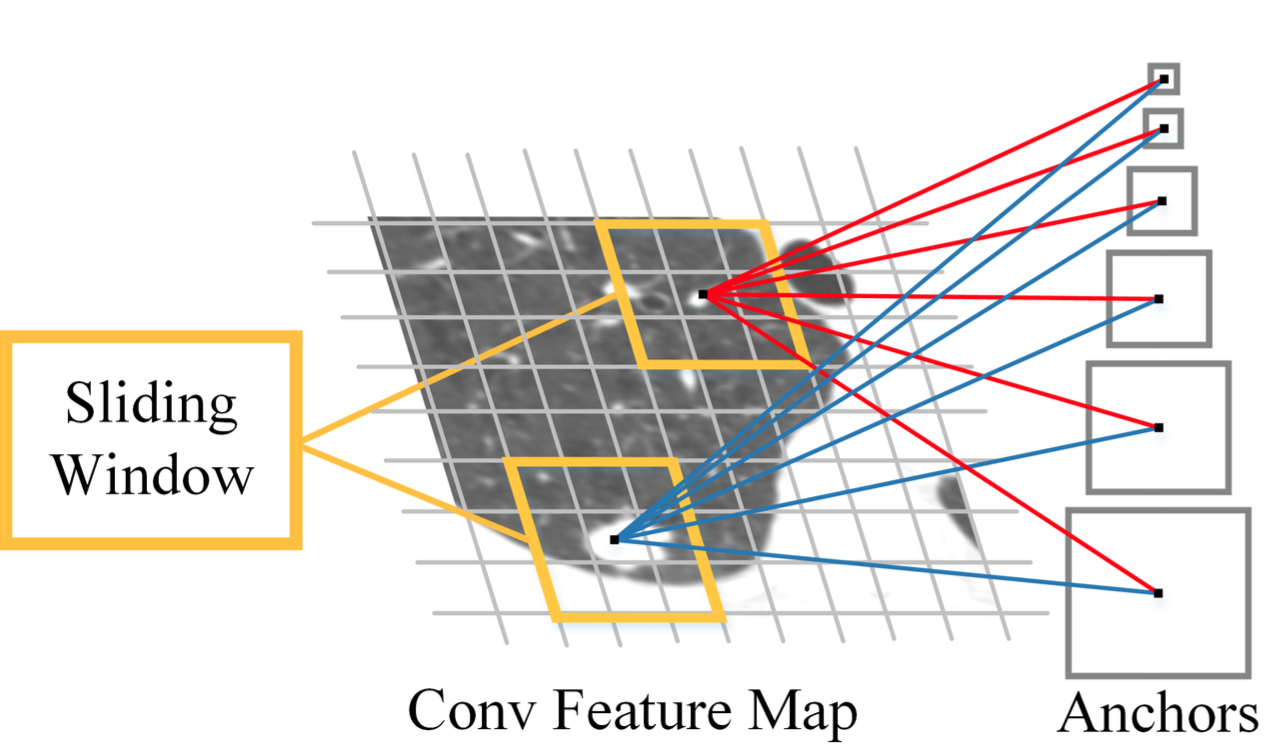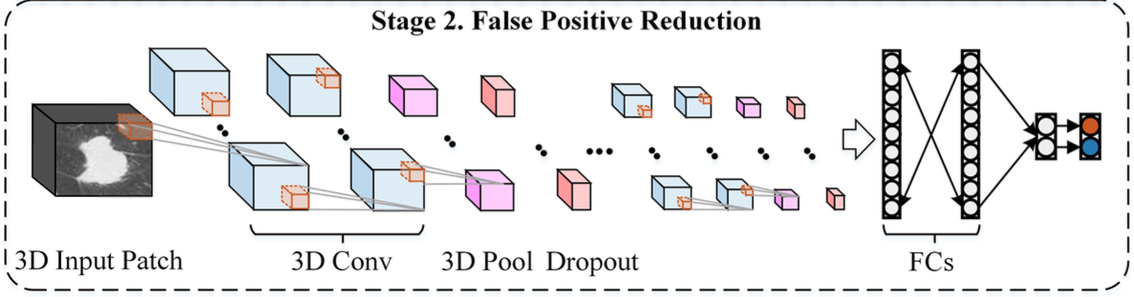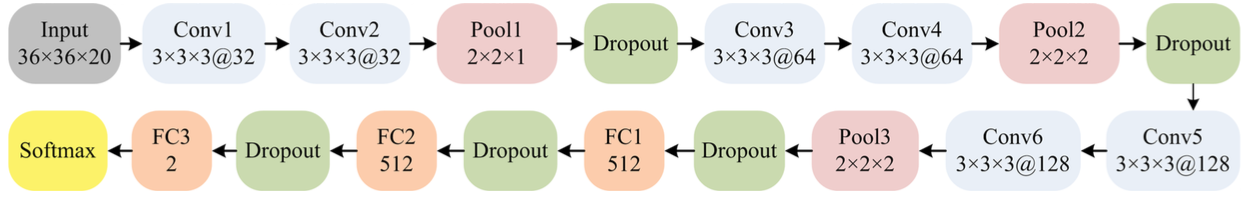# qfpxfd algorithm¶

## Summary¶

Kaggle solution Authors: Jia Ding, JunGao, WangDongLuna solution Authors: Jia Ding, Aoxue Li, Zhiqiang Hu and Liwei Wang The 4th place at the Data Science Bowl 2017 on the private leaderboard.

## Prerequisites¶

`Unknown`

Dependency Name Version
Language Python
ML engine Keras
ML backend Tensorflow

Dependency packages: `Unknown`

## Algorithm design¶

The algorithm itself consists of 2 steps:

• Candidate detection
• False Positive Reduction

### Preprocessing¶

The axial slices are used as inputs. For each axial slice in CT images, its two neighbors slices are concatenated in axial direction, and then rescaled into 600×600×3 voxels.

### Candidate Detection¶the architecture of the proposed candidate detection network is composed of two modules: a region proposal network (RPN) that aims to propose potential regions of nodules (also called Region-of-Interest (ROI)); a ROI classifier then recognizes whether ROIs are nodules or not. These two DCNNs share the same feature extraction layers.

The region proposal network takes an image as input and outputs a set of rectangular object proposals (i.e. ROIs), each with an objectness score. It is based on an original Faster R-CNN with a deconvolutional layer added.

To generate ROIs, a small network is slided over the feature map of the deconvolutional layer. At each sliding-window location, multiple ROIs are simultaneously predicted. The multiple ROIs are parameterized relative to the corresponding reference boxes, called anchors.With the ROIs extracted by RPN, a DCNN is developed to decide whether each ROI is nodule or not. A ROI Pooling layer is firstly exploited to map each ROI to a small feature map.

The ROI pooling works by dividing the ROI into a grid of sub-windows and then max-pooling the values in each sub-window into the corresponding output grid cell. Pooling is applied independently to each feature map channel as in standard max pooling. After ROI pooling layer, a fully-connected network, which is composed of two 4096-way fully-connected layers, then map the fixed-size feature map into a feature vector. A regressor and a classifier based on the feature vector then respectively regress boundingboxes of candidates and predict candidate confidence scores.

### False Positive Reduction Using 3D DCNN¶With the extracted nodule candidates, a 3D DCNN is utilized for false positive reduction. This network contains six 3D convolutional layers which are followed by Rectified Linear Unit (ReLU) activation layers, three 3D max-pooling lay- ers, three fully connected layers, and a final 2-way softmax activation layer to classify the candidates from nodules to none-nodules.As for inputs of the proposed 3D DCNN, each CT scan is firstly normalized with a mean of -600 and a standard deviation of -300. After that, for each candidate, the center of candidate is used as the centroid and then crop a 40 × 40 × 24 patch.

## Trained model¶

Usage instructions:`unknown`
`Unknown`